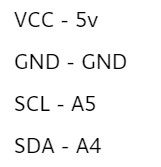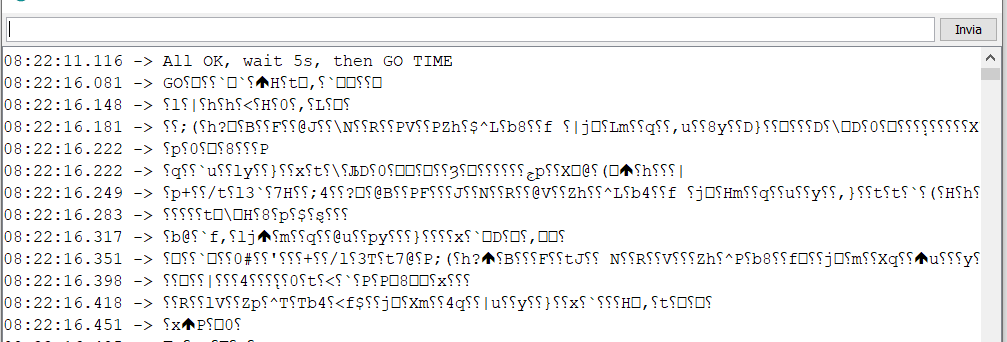Instantly share code, notes, and snippets.

# veproza/accelerometer.ino

Last active August 31, 2021 09:14
Accelerometer sampled at 1kHz, saved to SD card
This file contains bidirectional Unicode text that may be interpreted or compiled differently than what appears below. To review, open the file in an editor that reveals hidden Unicode characters. Learn more about bidirectional Unicode characters
 // Based on MPU-6050 Short Example Sketch // By Arduino User JohnChi // August 17, 2014 // Public Domain #include #include #include #include #define bufferLength 128 const int MPU=0x68; // I2C address of the MPU-6050 int second = floor(millis() / 1000); File myFile; volatile byte buf1[bufferLength]; volatile byte buf2[bufferLength]; byte toSend[bufferLength]; volatile uint8_t counter1; volatile uint8_t counter2; volatile bool secondBuffer = false; void setup(){ Wire.begin(); Wire.beginTransmission(MPU); Wire.write(0x6B); // PWR_MGMT_1 register Wire.write(0); // set to zero (wakes up the MPU-6050) Wire.endTransmission(true); Serial.begin(116400); while (!Serial) {} pinMode(10, OUTPUT); if (!SD.begin(10)) { Serial.println("initialization failed!"); return; } myFile = SD.open("test.txt", FILE_WRITE); Serial.println("All OK, wait 5s, then GO TIME"); delay(5000); Serial.print("GO"); Timer1.initialize(1000); Timer1.attachInterrupt(readData); } bool dead = false; volatile uint8_t fucks = 0; void loop() { second = floor(millis() / 1000); if(dead) { return; } if(second > 60 || fucks > 5) { if(!dead) { Serial.println("END"); Serial.println(fucks); myFile.close(); Serial.println("Closed"); dead = true; } return; } if(counter1 >= bufferLength or counter2 >= bufferLength) { noInterrupts(); if(counter1 >= bufferLength) { memcpy((char*)toSend, (char*)buf1, bufferLength); counter1 = 0; } else { memcpy((char*)toSend, (char*)buf2, bufferLength); counter2 = 0; } interrupts(); myFile.write(toSend,bufferLength); } } void readData(){ interrupts(); Wire.beginTransmission(MPU); Wire.write(0x3B); // starting with register 0x3B (ACCEL_XOUT_H) Wire.endTransmission(false); Wire.requestFrom(MPU,2,true); uint8_t acc_msb = Wire.read(); uint8_t acc_lsb = Wire.read(); noInterrupts(); uint16_t time = micros() % 50000; uint8_t time_msb = time >> 8; uint8_t time_lsb = time; if(secondBuffer == false) { if(counter1 == bufferLength) { fucks += 1; } else { buf1[counter1] = acc_msb; buf1[counter1 + 1] = acc_lsb; buf1[counter1 + 2] = time_msb; buf1[counter1 + 3] = time_lsb; counter1 += 4; if(counter1 == bufferLength) { secondBuffer = true; } } } else { if(counter2 == bufferLength) { fucks += 1; } else { buf2[counter2] = acc_msb; buf2[counter2 + 1] = acc_lsb; buf2[counter2 + 2] = time_msb; buf2[counter2 + 3] = time_lsb; counter2 += 4; if(counter2 == bufferLength) { secondBuffer = false; } } } }

### p4olo-90 commented Aug 31, 2021

Hi Veproza! Thank you for your help in the last post!
Now I am trying your code with arduino Nano + MPU_6050 connected via I2C.I want print the value of acceleration on "Serial Monitor" but something goes wrong like you can see in the picture:I have change your code just a little deleting all the line about the SD card function and adding the line below to print the value :
(the bundle rate is correctly setted)

` String myString = (char*)toSend; Serial.println(myString);`

Can you help me ?
Thanks

Below the complete code:

### _// Based on MPU-6050 Short Example Sketch

// By Arduino User JohnChi
// August 17, 2014
// Public Domain

#include <Wire.h>
#include <TimerOne.h>
#include <SD.h>
#include <SPI.h>

#define bufferLength 128

const int MPU=0x68; // I2C address of the MPU-6050

int second = floor(millis() / 1000);
File myFile;

volatile byte buf1[bufferLength];
volatile byte buf2[bufferLength];
byte toSend[bufferLength];

volatile uint8_t counter1;
volatile uint8_t counter2;

volatile bool secondBuffer = false;

void setup(){
Wire.begin();
Wire.beginTransmission(MPU);
Wire.write(0x6B); // PWR_MGMT_1 register
Wire.write(0); // set to zero (wakes up the MPU-6050)
Wire.endTransmission(true);
Serial.begin(115200);
while (!Serial) {}
pinMode(10, OUTPUT);
// if (!SD.begin(10)) {
// Serial.println("initialization failed!");
// return;
// }
//myFile = SD.open("test.txt", FILE_WRITE);
Serial.println("All OK, wait 5s, then GO TIME");
delay(5000);
Serial.print("GO");
Timer1.initialize(1000);
}

volatile uint8_t fucks = 0;
void loop() {
second = floor(millis() / 1000);
return;
}
if(second > 60 || fucks > 5) {
Serial.println("END");
Serial.println(fucks);
myFile.close();
Serial.println("Closed");
}
return;
}

if(counter1 >= bufferLength or counter2 >= bufferLength) {
noInterrupts();
if(counter1 >= bufferLength) {
memcpy((char*)toSend, (char*)buf1, bufferLength);
counter1 = 0;
} else {
memcpy((char*)toSend, (char*)buf2, bufferLength);
counter2 = 0;
}
interrupts();
// myFile.write(toSend,bufferLength);
// String myString = String(toSend);
String myString = (char*)toSend;

``````Serial.println(myString);
``````

}
}

interrupts();
Wire.beginTransmission(MPU);
Wire.write(0x3B); // starting with register 0x3B (ACCEL_XOUT_H)
Wire.endTransmission(false);
Wire.requestFrom(MPU,2,true);
noInterrupts();
uint16_t time = micros() % 50000;
uint8_t time_msb = time >> 8;
uint8_t time_lsb = time;

if(secondBuffer == false) {
if(counter1 == bufferLength) {
fucks += 1;
} else {
buf1[counter1] = acc_msb;
buf1[counter1 + 1] = acc_lsb;
buf1[counter1 + 2] = time_msb;
buf1[counter1 + 3] = time_lsb;
counter1 += 4;
if(counter1 == bufferLength) {
secondBuffer = true;
}
}
} else {
if(counter2 == bufferLength) {
fucks += 1;
} else {
buf2[counter2] = acc_msb;
buf2[counter2 + 1] = acc_lsb;
buf2[counter2 + 2] = time_msb;
buf2[counter2 + 3] = time_lsb;
counter2 += 4;
if(counter2 == bufferLength) {
secondBuffer = false;
}
}
}
}_

### veproza commented Aug 31, 2021

Hi,

so, here's the thing: this code is not particularly great for learning. It's a part of my thesis and the reasoning for what's happening inside is in that thesis, not in the code in form of comments (or at least a properly written clean code).

Anyway, the trouble is that what you're outputting to Serial is what I was writing to SD card. And to save on memory and bandwidth, I saved all the data in binary. That means that for an integer value of 60, I would write 0x3C to the SD card. However, the serial console tries to parse that as an ASCII text, outputting "<" (see ASCII table).

If you want to convert integer 60 to string "60", you'd need to call the function String with the integer as first parameter and "DEC" as second. Or pass in "DEC" as the second parameter of Serial.write, as it works much like the String function.

To further complicate matters, I stored the values in RAM buffers, so that I could write to SD cards in batches (to save on the write count and therefore the speed of SD card degradation). And also my code adds a timestamp to each datapoint so the data could be reconstructed.

Personally, for debugging, I'd rather rewrite the data collection part of the code

```void readData(){
// interrupts(); not necessary
Wire.beginTransmission(MPU);
Wire.write(0x3B);  // starting with register 0x3B (ACCEL_XOUT_H)
Wire.endTransmission(false);
Wire.requestFrom(MPU,2,true);